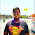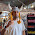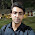## Saturday, June 6, 2015

### Linear Regression with single/multiple Variables Assignment Solutions : coursera.org (Machine Learning) Week 2

My solutions to the exercises:

Part 1 : Warm up exercise

```function A = warmUpExercise()
%WARMUPEXERCISE Example function in octave
%   A = WARMUPEXERCISE() is an example function that returns the 5x5 identity matrix

A = [];
% ============= YOUR CODE HERE ==============
% Instructions: Return the 5x5 identity matrix
%               In octave, we return values by defining which variables
%               represent the return values (at the top of the file)
%               and then set them accordingly.

A = eye(5);
% ===========================================

end```

Part 2 : Compute cost for one variable

```function J = computeCost(X, y, theta)
%COMPUTECOST Compute cost for linear regression
%   J = COMPUTECOST(X, y, theta) computes the cost of using theta as the
%   parameter for linear regression to fit the data points in X and y

% Initialize some useful values
m = length(y); % number of training examples

% You need to return the following variables correctly
J = 0;

% ====================== YOUR CODE HERE ======================
% Instructions: Compute the cost of a particular choice of theta
%               You should set J to the cost.
predictions = X*theta;
squaredError = (predictions-y) .^ 2;
J = (1/(2*m))*(sum(squaredError));

% =========================================================================

end```

Part 3: Gradient descent for one variable

```function [theta, J_history] = gradientDescent(X, y, theta, alpha, num_iters)
%   taking num_iters gradient steps with learning rate alpha

% Initialize some useful values
m = length(y); % number of training examples
J_history = zeros(num_iters, 1);
for iter = 1:num_iters

% ====================== YOUR CODE HERE == ====================
% Instructions: Perform a single gradient step on the parameter vector
%               theta.
%
% Hint: While debugging, it can be useful to print out the values
%       of the cost function (computeCost) and gradient here.
%
predictions = X*theta;
errors = predictions-y;
mulAndSum = errors' * (X);
right = (alpha*mulAndSum)/m;
theta = theta-(right');
% ============================================================

% Save the cost J in every iteration
J_history(iter) = computeCost(X, y, theta);

end

end
```

Part 4 : Feature normalization

```function [X_norm, mu, sigma] = featureNormalize(X)
%FEATURENORMALIZE Normalizes the features in X
%   FEATURENORMALIZE(X) returns a normalized version of X where
%   the mean value of each feature is 0 and the standard deviation
%   is 1. This is often a good preprocessing step to do when
%   working with learning algorithms.

% You need to set these values correctly
X_norm = X;
mu = zeros(1, size(X, 2));
sigma = zeros(1, size(X, 2));

% ====================== YOUR CODE HERE ======================
% Instructions: First, for each feature dimension, compute the mean
%               of the feature and subtract it from the dataset,
%               storing the mean value in mu. Next, compute the
%               standard deviation of each feature and divide
%               each feature by it's standard deviation, storing
%               the standard deviation in sigma.
%
%               Note that X is a matrix where each column is a
%               feature and each row is an example. You need
%               to perform the normalization separately for
%               each feature.
%
% Hint: You might find the 'mean' and 'std' functions useful.
%

for i = 1:size(X)(2) mu(1, i) = mean(X(:,[i:i]));end
for i = 1:size(X)(2) sigma(1, i) = std(X(:, [i:i]));end
for i = 1:size(X)(1)
for j = 1:size(X)(2)
X_norm(i, j) = ((X(i, j)-mu(1, j))/sigma(1, j));
end
end
% ============================================================

end```

Part 5: Compute Cost for Multiple Variables

```function J = computeCostMulti(X, y, theta)
%COMPUTECOSTMULTI Compute cost for linear regression with multiple variables
%   J = COMPUTECOSTMULTI(X, y, theta) computes the cost of using theta as the
%   parameter for linear regression to fit the data points in X and y

% Initialize some useful values
m = length(y); % number of training examples

% You need to return the following variables correctly
J = 0;

% ====================== YOUR CODE HERE ======================
% Instructions: Compute the cost of a particular choice of theta
%               You should set J to the cost.
predictions = X*theta;
squaredError = (predictions-y) .^ 2;
J = (1/(2*m))*(sum(squaredError));

% =========================================================================

end```

Part 6: Gradient Descent for Multiple Variables

```function [theta, J_history] = gradientDescentMulti(X, y, theta, alpha, num_iters)
%   taking num_iters gradient steps with learning rate alpha

% Initialize some useful values
m = length(y); % number of training examples
J_history = zeros(num_iters, 1);
for iter = 1:num_iters

% ====================== YOUR CODE HERE == ====================
% Instructions: Perform a single gradient step on the parameter vector
%               theta.
%
% Hint: While debugging, it can be useful to print out the values
%       of the cost function (computeCost) and gradient here.
%
predictions = X*theta;
errors = predictions-y;
mulAndSum = errors' * (X);
right = (alpha*mulAndSum)/m;
theta = theta-(right');
% ============================================================

% Save the cost J in every iteration
J_history(iter) = computeCostMulti(X, y, theta);

end

end```

Part 7: Normal Equations

```function [theta] = normalEqn(X, y)
%NORMALEQN Computes the closed-form solution to linear regression
%   NORMALEQN(X,y) computes the closed-form solution to linear
%   regression using the normal equations.

theta = zeros(size(X, 2), 1);

% ====================== YOUR CODE HERE ======================
% Instructions: Complete the code to compute the closed form solution
%               to linear regression and put the result in theta.
%

% ---------------------- Sample Solution ----------------------

theta = (pinv(X'*X)*X'*y);

% -------------------------------------------------------------

% ============================================================

end```

1.2.3.